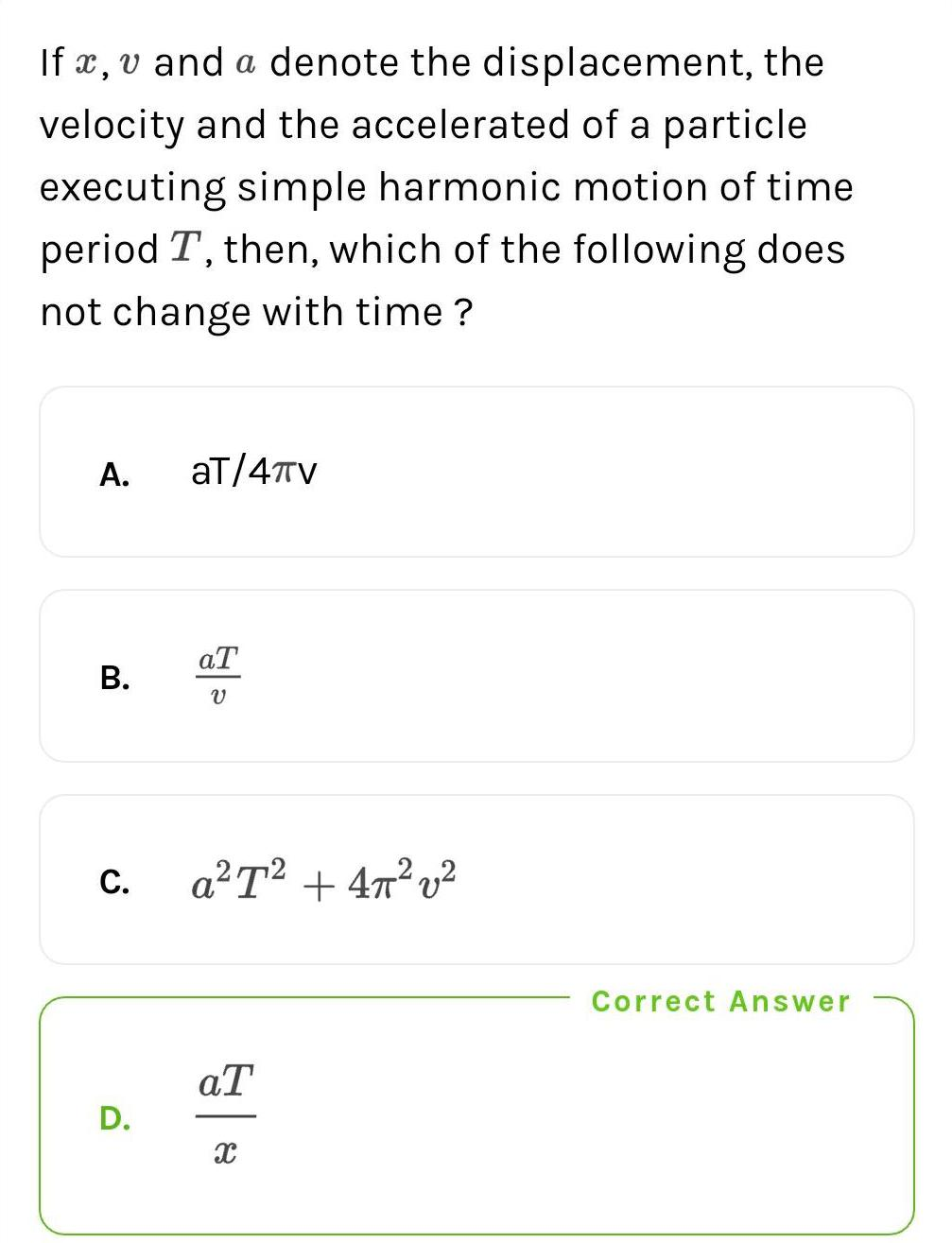Question:

# If x, v and a denote the displacement, the velocity and theIf x, v and a denote the displacement, the velocity and the accelerated of a particle executing simple harmonic motion of time. period T, then, which of the following does not change with time? A. aT/4πV B.aT/V C.a²T² + 4π²v² D.aT/X